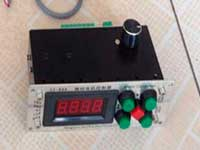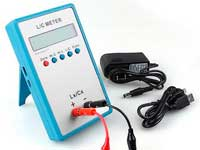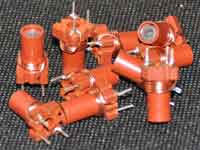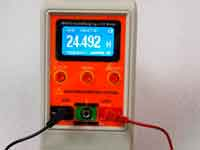How to calculate inductance? - 5.0 out of 5 based on 2 votes
Rating 5.00 (2 Votes)

How to calculate inductance?We can use different methods to calculate the inductance using numerical methods or handbook formulas. They can be conditionally divided into three levels - high, medium and low level.

High level involves the use of programs having a common name - electromagnetic simulators. For example, Comsol Multisystems with RF module, Ansys HFSS, etc. Their work is based on the differential Maxwell's equations for the electromagnetic field providing the boundary conditions.
Advantages: accurate calculation of inductance and other parameters of the coil with any geometry of winding in any frequency range.
Disadvantages: these programs are quite complex and require prior learning, require large computational resources, the calculation takes a long time. Can be used for professional work or if the inductor is used at or above the self-resonance frequency.

The medium level is based on the simplified model of the inductor introduced by J.C.Maxwell.
Advantages: acceptable calculation accuracy for radio-enthusiasts practice, the opportunity of using in simple programs, low demands on the processing power of the computer.
Disadvantages: less accurate calculations than the high level; the calculation isn't possible for any winding geometry and maybe only in the frequency range that does not exceed 60-70% of the self-resonant frequency (or rather 1-resonance) of the coil.

The low level is based on simple handbook formulas. These formulas are based on the simplification of medium level formulas or on the basis of a set of measurements of the actual coils.
Advantages: simple calculation, undemanding to resources of the computer

Disadvantages: the formulas work only with a restricted geometry of the winding and at frequencies much lower than the self-resonance frequency.

More about medium level calculation that Col32 uses... The great physicist J.C. Maxwell has shown in the late XIX century in his famous work - "A Treatise on Electricity and Magnetism."  that the mutual inductance between two infinitely thin circular coaxial conductors can be calculated as follows:Where•     M - mutual inductance;
•     r1, r2 - radii of the two circular filaments;
•     x - the distance between the centers of the circles bounded by these filaments;
•     K, E - elliptic integrals of the first and second kind;

A numerical method for the calculation of Maxwell's formula reduced to numerical methods for solving elliptic integrals.

By using Maxwell's equation can be calculated the inductance of a single-layer, multi-layer or flat coil and the mutual inductance of two separate coils. Errors related to the coaxial circular filaments approximation (in fact we deal the round wire helix) can be reduced through additional corrections.

Сomments from anonymous guests are enabled with moderation.

Coil Winding MachineUS \$187.53
The system consists of individual components including a controller, a stepping motor, a foot switch, power adapter and output shaft. This design makes it very easy for you to integrate the system into your own project.

LC Meter LC200AUS \$37.99
Measuring Capacitance Range:
0.01 pF - 100mF
Measuring Inductance Range:
0.001 uH - 100H
Measurement accuracy:1%.

RF InductorsUS \$6.43
Variable Ferrite Core Inductor coil set 3.5Turns 25-100MHZ

Auto Ranging LCR MeterUS \$63.07
Meter can measure 0.00pF to 100.00mF capacitance, 0.000uH to 100.00H inductance and 0.00? to 10.000M? resistance. Very suitable for small value capacitor and inductor measurement.